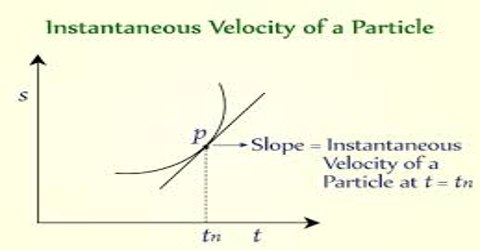Physics

# Instantaneous Velocity Related to MotionInstantaneous velocity or velocity:

If the time interval approaches zero, the rate of change of displacement with time is called instantaneous velocity or simply velocity.

Instantaneous velocity means the velocity of a body at a particular time. In order to find the instantaneous velocity of a body, at an instant, it is essential to know the velocity just before and just after that instant. This time interval at must be exceedingly small (almost zero). This is determined similarly to average velocity, but we narrow the period of time so that it approaches zero. If an object has a standard velocity over a period of time, its average and instantaneous velocities may be the same.That means, when tune interval tends to zero, rate of change of displacement for that time interval is called instantaneous velocity.

So, instantaneous one-dimensional velocity: vx = lit∆t→0 ∆x/∆t = dx/dt.

From the above discussion instantaneous velocity may be defined as:

If the time interval tends to zero, limiting value of the velocity is called instantaneous velocity or velocity.

To calculate instantaneous speed, we need to divide part of the total distance traveled by time. However, we don’t want to use the distance of the entire trip, because that will give us average speed.